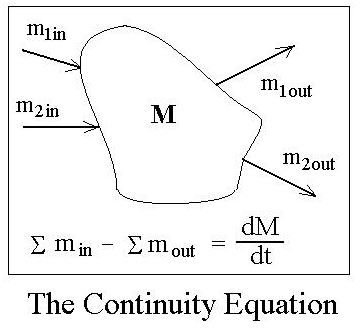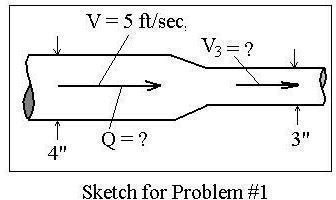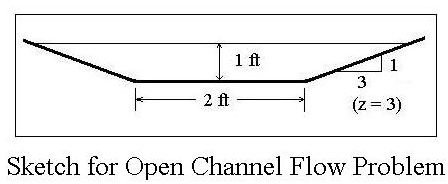# Conversion of Linear Velocity to Volumetric Flow Rate or to Mass Flow Rate

Page content

## Commonly Used Parameters for Fluid Flow Rate

Three different parameters commonly used as measures of fluid flow rate for either pipe flow or open channel flow are volumetric flow rate, mass flow rate, and average velocity. Volumetric flow rate is the one most widely used for liquids. As implied by the name, its units will be volume of flow per unit time. For gases, temperature and pressure have significant effects on the gas density, and thus on the volumetric flow rate, so mass flow rate is sometimes used instead of volumetric flow rate for gases. For flow of fluids in pipes, ducts, or open channels, the velocity will not be constant over the cross-sectional area of flow, yet some measure of the fluid velocity is often of interest. The velocity parameter most widely used is the average velocity, defined to be the volumetric flow rate divided by the cross-sectional area of flow. Summarizing these three parameters with the symbols used for them and typical units are:

• Volumetric Flow Rate; Symbol: Q; Units: ft3/sec, gallons per min, m3/sec, etc
• Mass Flow Rate; Symbol: m; Units: lb/hr, kg/min, etc
• Average Velocity; Symbol: V; Units: ft/sec or m/sec

## Equations for Conversion of Linear Velocity to Volumetric Flow Rate and to Mass Flow Rate

The equation for conversion of linear velocity (average velocity) to volumetric flow rate comes from the definition for average velocity mentioned above:

V = Q/A, or Q = VA,

where V is the average velocity and A is the cross-sectional area of the fluid perpendicular to flow.

The mass flow rate is simply the fluid density multiplied by the volumetric flow rate, or:

m = ρQ = ρVA, where ρ = the density of the fluid. This is summarized in the figure at the left.

## The Fluid Mechanics Continuity Equation

The basic principle of conservation of mass as applied to a fluid system is typically called the continuity equation. In its most generalform, the unsteady state, the compressible flow continuity equation states that the total rate of mass flow into a system minus the total rate of mass flow out of that system at any time must equal the rate of change of mass in the system. Written as an equation this is:

Σmin -Σmout - dM/dt, where M is the mass of fluid in the system.

Many fluid flow systems operate under steady state flow conditions. That is, the total mass flow into the system and total mass flow out of the system remain constant with time. In that case, the amount of mass in the system doesn’t change, so dM/dt = zero, and the steady state form of the compressible flow continuity equation becomes:

Σmin = Σmout or ΣρQin = ΣρQout or ΣρVAin = ΣρVAout

Another simplification of the continuity equation is its application to incompressible flow. In fluid mechanics the term, ‘incompressible flow,’ doesn’t really refer to a fluid that cannot be compressed. Rather it refers to a situation in which the density of a fluid is not changing for a given set of fluid flow conditions. In general, the flow of liquids can be treated as incompressible flow and many cases of flowing gases must be treated as compressible flow. For incompressible flow, the density, ρ, remains constant, so the incompressible flow continuity equation becomes:

ΣQin = ΣQout or ΣVAin = ΣVAout.

## Example Calculations

Problem Statement #1: Water flow through a 4 inch diameter pipe approaching a reducing fitting withan average velocity of 5 ft/sec. The fitting reduces to a 3 inch diameter pipe. i) What is the volumetric flow rate through this pipe? and ii) What is the average velocity of the water in the 3 inch diameter pipe? See the sketch at the right.

Solution: i) Conversion of linear velocity to volumetric flow rate:

Q = VA = V(πD2/4) = (5 ft/sec)[π(4/12)2/4)ft], or Q = 0.4363 ft3/sec

ii) The volumetric flow rate through the 3" pipe must be the same, so:

(V3)[π(3/12)2]/4 = 0.436 ft3/sec. Solving for V3 gives: V3 = 8.88 ft/secProblem Statement #2: A trapezoidal open channel with bottom width of 2 ft and side slopes of horiz:vert = 3:1 (as shown in the diagram at the left), carries 20 ft3/sec when it is flowing 1 ft deep. What is the average velocity in the channel under these conditions?

Solution: From the article, “Calculation of Hydraulic Radius for Open Channel Flow,” the trapezoidal cross-sectional area of flow can be calculated from: A = by + zy2, where b is the channel bottom width, y is the depth of flow, and z is horz:vert side slope. Thus A = (2)(1) + 3(12) = 5 ft2, and V = Q/A = (20 ft3/sec)/5 ft2), or V = 4 ft/sec.